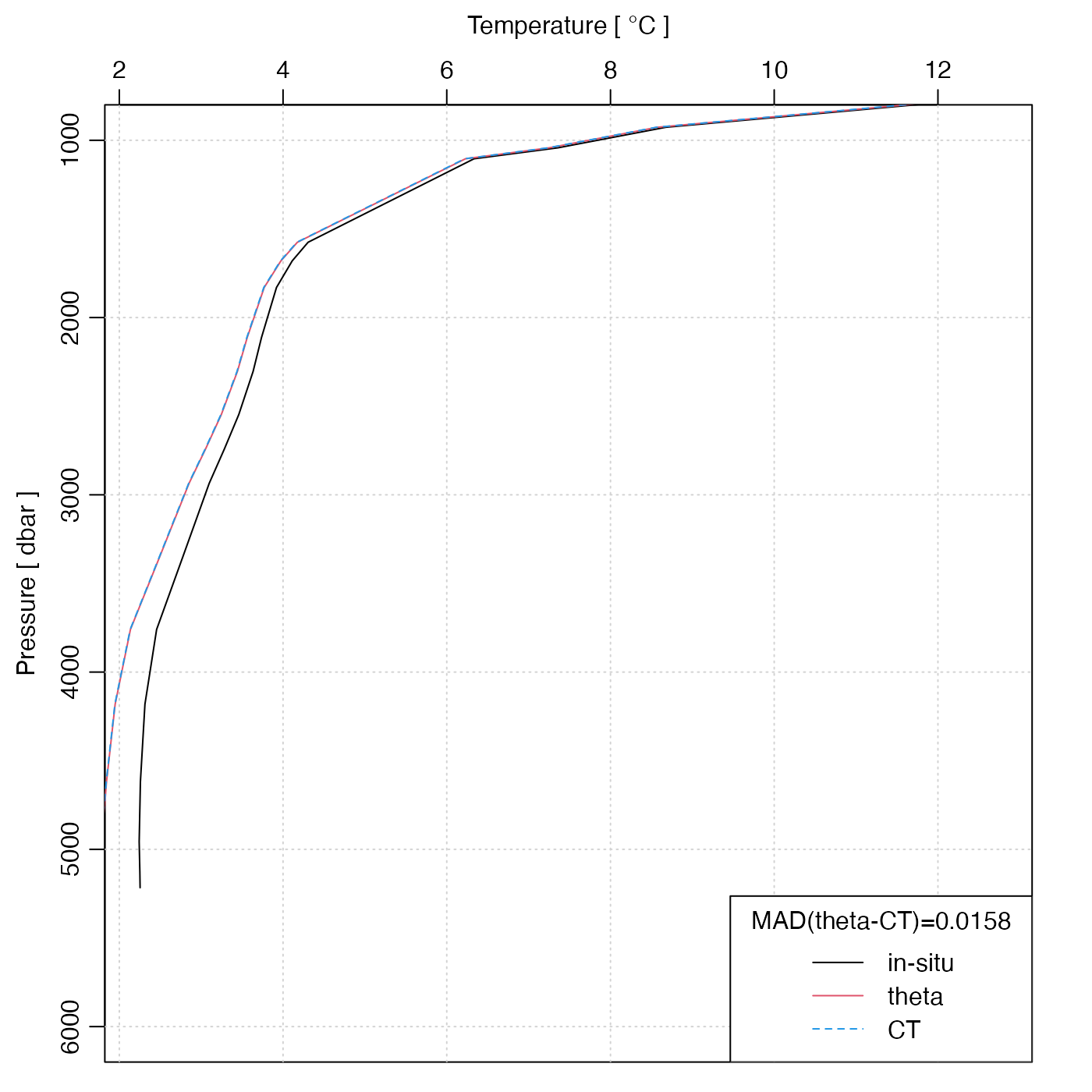Compute the potential temperature of seawater, denoted $$\theta$$ in the UNESCO system, and pt in the GSW system.

swTheta(
salinity,
temperature = NULL,
pressure = NULL,
referencePressure = 0,
longitude = NULL,
latitude = NULL,
eos = getOption("oceEOS", default = "gsw")
)

## Arguments

salinity

either salinity (PSU) (in which case temperature and pressure must be provided) or an oce object (in which case salinity, etc. are inferred from the object).

temperature

in-situ temperature ($$^\circ$$C), defined on the ITS-90 scale; see “Temperature units” in the documentation for swRho(), and the examples below.

pressure

pressure (dbar)

referencePressure

reference pressure (dbar)

longitude

longitude of observation (only used if eos="gsw"; see “Details”).

latitude

latitude of observation (only used if eos="gsw"; see “Details”).

eos

equation of state, either "unesco" (references 1 and 2) or "gsw" (references 3 and 4).

## Value

Potential temperature ($$^\circ$$C) of seawater, referenced to pressure referencePressure.

## Details

Different formulae are used depending on the equation of state. If eos is "unesco", the method of Fofonoff et al. (1983) is used (see references 1 and 2). Otherwise, swTheta uses gsw::gsw_pt_from_t() from the gsw package.

If the first argument is a ctd or section object, then values for salinity, etc., are extracted from it, and used for the calculation, and the corresponding arguments to the present function are ignored.

Other functions that calculate seawater properties: T68fromT90(), T90fromT48(), T90fromT68(), computableWaterProperties(), locationForGsw(), swAbsoluteSalinity(), swAlphaOverBeta(), swAlpha(), swBeta(), swCSTp(), swConservativeTemperature(), swDepth(), swDynamicHeight(), swLapseRate(), swN2(), swPressure(), swRho(), swRrho(), swSCTp(), swSR(), swSTrho(), swSigma0(), swSigma1(), swSigma2(), swSigma3(), swSigma4(), swSigmaTheta(), swSigmaT(), swSigma(), swSoundAbsorption(), swSoundSpeed(), swSpecificHeat(), swSpice(), swSstar(), swTFreeze(), swTSrho(), swThermalConductivity(), swViscosity(), swZ()

Dan Kelley

## Examples

library(oce)
# Example 1: test value from Fofonoff et al., 1983
stopifnot(abs(36.8818748026 - swTheta(40, T90fromT68(40), 10000, 0, eos = "unesco")) < 0.0000000001)

# Example 2: a deep-water station. Note that theta and CT are
# visually identical on this scale.
data(section)
stn <- section[["station", 70]]
plotProfile(stn, "temperature", ylim = c(6000, 1000))
lines(stn[["theta"]], stn[["pressure"]], col = 2)
lines(stn[["CT"]], stn[["pressure"]], col = 4, lty = 2)
legend("bottomright",
lwd = 1, col = c(1, 2, 4), lty = c(1, 1, 2),
legend = c("in-situ", "theta", "CT"),
title = sprintf("MAD(theta-CT)=%.4f", mean(abs(stn[["theta"]] - stn[["CT"]])))
)